### Geometrical Shapes - Worksheets

CBSE Worksheet-01

Class - IV Mathematics – Geometrical Shapes

Time Allowed: 30 minutes

1. Fill in the blanks with suitable words:
1. A brick has ________ edges.
2. Each ray of an angle is called a/an _________ of the angle.
3. The sum of length of line segments of a figure is called its _________.
4. The standard unit of angles is ________.
5. A _______ has no length, breadth or height.
2. State whether each of the following statement is True or False:
1. An angle equal to 90${}^{\circ }$ is called an acute angle.
2. An angle greater than 180${}^{\circ }$ is called a reflex angle.
3. circle is a closed figure.
4. Vertex of the given angle is A.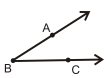5. An angle which measures 90o is called a straight angle.
3. Diameter of a circle is _________the length of its radius.
1. Thrice
2. Equal
3. Twice
4. Half
4. In a rectangle, the pairs of ______ sides are parallel and equal.
1. None of these
2. Equal
3. Twice
4. Opposite
5. What will be the perimeter of the triangle, whose sides are 20 cm, 25 cm, and 40 cm?
1. 45 cm
2. 120 cm
3. 85 cm
4. 150 cm
6. The numbers on the opposite faces of this box add up to 7. Which number was on the opposite side of 5?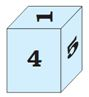1. 3
2. 2
3. 4
4. 1
7. Find the circumference of a circle with a radius of a circle 21cm.
1. 132 cm
2. 44 cm
3. 154 cm
4. 56 cm
8. Match the following:

 (a) Equilateral triangle (i) One angle is the right angle (b) Isosceles triangle (ii) All three sides have equal measure (c) Scalene triangle (iii) Two sides have equal measure (d) Right angle triangle (iv) Three sides have different measure
9. What can be used to measure an angle?

10. Name the radius and diameter of the given circle.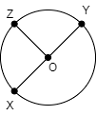11. Answer the following question with the help of the figure.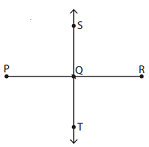1. Name two line segments = ______
2. Name two opposite rays = ______
12. How many line segments are there in the following figure. Also, write name of all the line segments.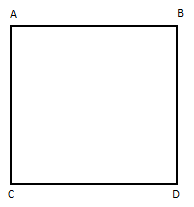13. Identify the shape using given properties and draw its shape. Three sides shape with two equal sides and one angle equal to ${90}^{\circ }$.

14. The diameter of the circle is given. Find the radius of the circle.
10 cm, 16 cm,  8 cm,  22 cm

15. Find the perimeter of the following:

1. If sides of the triangle are 2 cm, 3 cm, and 5 cm
2. If one side of the equilateral triangle is 5 cm
3. If sides of the quadrilaterals are 4 cm, 6 cm, 2 cm, and 1 cm
4. If one side of the square is 10 cm
16. Measure the following angles. determine the type of the angles.

1.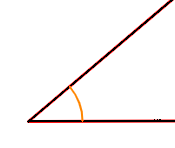2.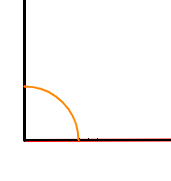CBSE Worksheet-01
Class - IV Mathematics – Geometrical Shapes

Solution

1. Fill in the blanks with suitable words:
1. 12. An edge is a particular type of line segment joining two vertices in a closed shape.
2. Side. An angle is the figure formed by two rays, called the sides of the angle.
3. Perimeter. Sum of the lengths of all line segments of any closed figure is called its perimeter.
5. Line.
A line extends indefinitely in a single dimension. Its length has no limit, it is infinite and it has no width or height.
2. State whether each of the following statement is True or False:
1. False,
An angle equal to 90${}^{\circ }$ is called a right angle.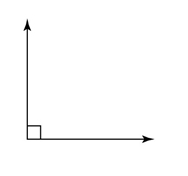2. True, An angle greater than 180${}^{\circ }$ is called a reflex angle.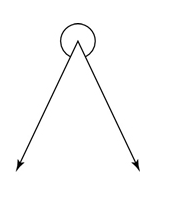3. True. Circle is a closed figure.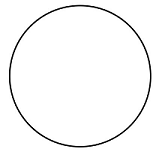4. False, Vertex of the given angle is B.
5. False. An angle which measures 90o is called a right angle.
3. (c) Twice
Explanation: A  diameter of a circle passes through the centre of the circle and touching two points on its edge.
4. (d) Opposite
Explanation: In a rectangle.
1. the opposite sides are parellel and equal.

2. the opposite angles are equal.

5. (c) 85 cm
Explanation: perimeter of triangle = A+B+C
(A, B and C are length of each sides of the triangle)
perimeter = 20 +25 + 40 = 85
6. (b) 2
Explanation: The number on the opposite faces of the box .when added it is 7
Example: if it is 4 on one side the opposite side will have number 3
4+3=7
, so when we see number 5, the opposite side of 5 will be 2
5+2=7
7. (a) 132 cm
Explanation: If the radius is 21cm than the diameter = 21 $×$ 2=42
C = $\pi$ d
C = $\pi$ (42) cm
C = (22/7) (42) cm
C = 22 $×$ 6 cm
C = 132 cm
8. (a) – (ii), (b) – (iii), (c) – (iv), (d) – (i)

1. In a triangle, all three sides have equal measure than it is called an equilateral triangle
2. In a triangle, two sides have equal measure than it is called an Isosceles triangle.
3. In a triangle, all three sides have different measure than it is called a Scalene triangle
4. In a triangle, if one angle is right angle than it is called a right angle triangle.
9. A protractor is used to measure an angle.

10.$\overline{OX}$$\overline{OY}$ and $\overline{OZ}$
2. DIAMETER
$\overline{XY}$
1. Name two line segments = $\overline{PR}$ & $\overline{ST}$
2. Name two opposite rays = $\stackrel{\to }{QS}$ & $\stackrel{\to }{QT}$

11.Numbers of line segments = 4
Name =  and

• It has three sides. $⇒$ means it is a triangle
• it has two equal sides.$⇒$ means isosceles triangle
• one angle is 90${}^{\circ }$$⇒$ means isosceles right angle triangle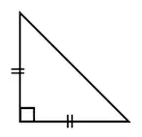12. Radius of the circle = $\frac{diameter}{2}$

1. Diameter of circle = 10 cm
Radius of the circle = $\frac{10}{2}$ = 5 cm
2. Diameter of circle = 16 cm
Radius of the circle = $\frac{16}{2}$ = 8 cm
3. Diameter of circle = 8 cm
Radius of the circle = $\frac{8}{2}$ = 4 cm
4.  Diameter of circle = 22 cm
Radius of the circle = $\frac{22}{2}$ = 11 cm
13. Perimeter = sum of all the sides

1. If sides of the triangle are 2 cm, 3 cm, and 5 cm
Perimeter = 2 cm + 3 cm + 5 cm = 10 cm
2. One side of the equilateral triangle is 5 cm
All the sides of the equilateral triangle are equal.
Perimeter = 5 cm + 5 cm + 5 cm = 15 cm
3. If sides of the quadrilaterals are 4 cm, 6 cm, 2 cm and 1 cm
Perimeter = 4 cm + 6 cm + 2 cm + 1 cm = 13 cm
4. If one side of the square is 10 cm
All the sides of the square are equal.
Perimeter = 10 cm + 10 cm + 10 cm + 10 cm = 40 cm

1.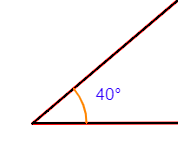Measure of an angle = ${40}^{\circ }$
Here, the measure of an angle is ${40}^{\circ }$, so it is an acute angle.
{If the measure of an angle is less than ${90}^{\circ }$ then it is an acute angle.}

2.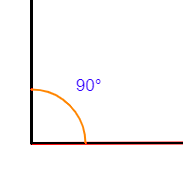Measure of an angle = ${90}^{\circ }$
Here, the measure of an angle is ${90}^{\circ }$, so it is a right angle.
{If the measure of an angle is equal to ${90}^{\circ }$ then it is a right angle.}